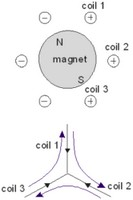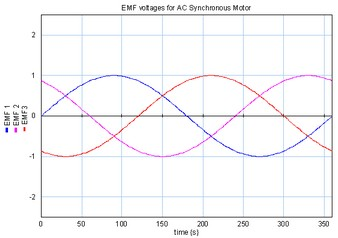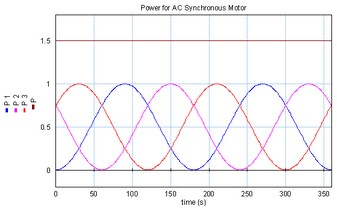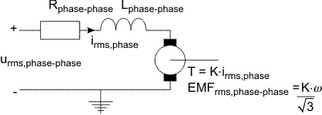﻿ 20-sim webhelp > Toolboxes > Mechatronics Toolbox > Servo Motor Editor > Theory > Basic Principles > AC synchronous motor

# AC synchronous motor

Navigation:  Toolboxes > Mechatronics Toolbox > Servo Motor Editor > Theory > Basic Principles >

# AC synchronous motorAC synchronous motors use the same principle as Brushless DC-motors. The coils are also mounted in the stator with a spatial displacement of 120°.The coils and magnets are designed so that a sinusoidal torque function Kc(q) is found as shown below:To get a constant torque each coil current is a sinusoidal wave as shown in the figure below.As can be seen from the graph, the resulting torque is constant and one and a half times the current times the amplitude of the coil function:To get an equivalent of the coil model we can write this equation as:We have to realize that here i is not a constant current anymore but the root mean square coil current! Because the coil current is the current of one phase in literature i is mostly denoted as the rms phase current. It is the rms current that can be measured at each of the three terminals of the motor.

In a derivation that goes beyond the scope of this introduction (see Compter, 2004), it can be shown that the electromotive force can be found as:Here the electromotive force is defined as the induced rms voltage between two phases. The resulting motor model is shown below:The voltage of the motor model is mostly denoted as the rms phase to phase voltage. It is the rms voltage that can be measured between any pair of two terminals. The phase to phase resistance and phase to phase inductance are defined equivalent as the resistance and inductance that could be measured between any pair of two terminals.

## Limits

AC synchronous motors have no mechanical commutation, and therefore do not suffer from the limits caused by mechanical commutation. Consequently AC synchronous motors can run at much higher speeds and are not limited by a maximum voltage. The speed of AC synchronous motors is only limited by the bearings.

Just like brush DC motors the magnets impose an upper limit for the current and torque in a AC synchronous motor.